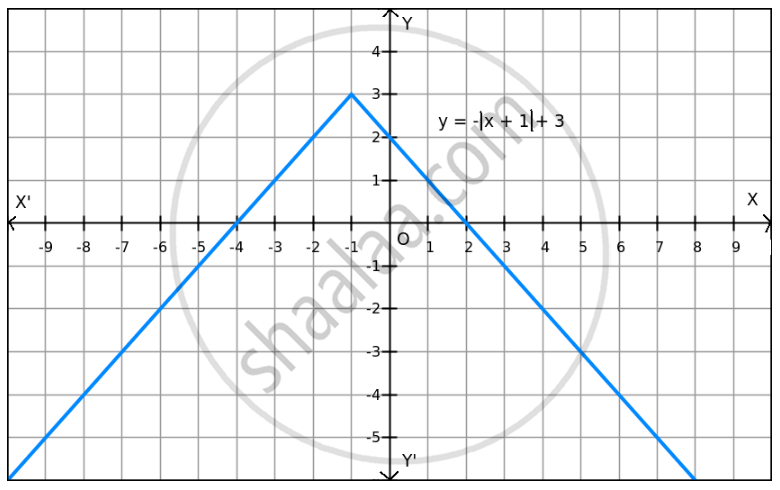PUC Karnataka Science Class 12Department of Pre-University Education, Karnataka
Share

F (X) = − | X + 1 | + 3 on R . - PUC Karnataka Science Class 12 - Mathematics

Question

f (x) = $-$ | x + 1 | + 3 on R .

Solution

Given: f(x) =$- \left| x + 1 \right|$ + 3

Now,

$- \left| x + 1 \right| \leq 0$ for all x $\in$ R.

$\Rightarrow$ f(x) = $- \left| x + 1 \right|$ + 3 $\leq$ 3 for all x $\in$ R
$\Rightarrow$ f(x) $\leq$ 3 for all x $\in$ RThe maximum value of f is attained when

$\left| x + 1 \right| = 0 .$

$\Rightarrow x = - 1$

Therefore, the maximum value of f at x = -1 is 3.

Since f(x) can be reduced, the minimum value does not exist, which is evident in the graph also.

Hence, the function f does not have a minimum value.

Is there an error in this question or solution?

Video TutorialsVIEW ALL 

Solution F (X) = − | X + 1 | + 3 on R . Concept: Graph of Maxima and Minima.
S